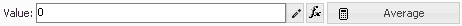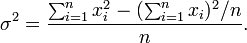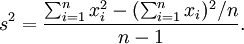﻿ Gauge Formulas
Nevron Vision for SharePoint Documentation
Gauge Formulas

Gauge formulas represent aggregate functions on sets of numeric values. Gauge formulas are applied to gauge properties extracted from gauge part data cells. Currently gauge formulas can be applied to the following gauge element properties:

• Axis Min
• Axis Max
• Pointer Value
• Range Begin
• Range End
• Numeric Display Value

In the visual interface, a gauge formula is always packed with an expression editor:The expression is stored in the gauge part data cell of the respective gauge element. The expression is evaluated by the Nevron Pivot Engine for each leaf category and produces a list of values. The gauge formula then extracts a single value from this list. See The Gauge Data Model for a complete discussion. Following is a description of the currently available gauge formulas:

Formula Description Options Example
Min Returns the minimal value from the set None Min(25, 10, 30) = 10
Max Returns the maximum value from the set None Max(25, 10, 30) = 30
Average Returns the average value in the set. Computed as SUM/COUNT. None Average(25, 10, 30) = 21.66
Sum Returns the sum of the values in the set. None Sum(25, 10, 30) = 65
First Returns the first value from the set. None First(25, 10, 30) = 25
Last Returns the last value from the set. None Last(25, 10, 30) = 30
Count Returns the count of value in the set. None Count(25, 10, 30) = 3
Median Returns a value which splits the set in half. Half of the values are smaller than the median value and half are larger. None Median(25, 10, 30) = 25
Median(25, 10, 20, 30) = 22.5
Percentile Returns a value which splits the set at arbitrary percent. {Percent count} of the values are smaller than this value and the other ones are larger. Percentile50 is equivalent to the Median formula. You can use the percentile to compute quartiles and deciles. 1 quartile is equivalent to Percentile25. 1 decile is equal to Percentile10. Percent

Percent = 25
Percentile(25, 10, 20, 30) = 15

Percent = 75
Percentile(25, 10, 20, 30) = 27.5

Variance

The variance is used to measure the tendency of the values in the set to deviate from the average.

If Entire Population is True, it is computed by this formula:If Entire Population is False (sample variance), it is computed by this formula:Entire Population EntirePopulation = true
Variance(4, 7, 13, 16) = 22.5

EntirePopulation = false
Variance(4, 7, 13, 16) = 30
StdDev

Returns the standard deviation of the values in the set. It is computed as the positive square root of the variance.

Entire Population EntirePopulation = true
StdDev(4, 7, 13, 16) = 4.74

EntirePopulation = false
StdDev(4, 7, 13, 16) = 5.48All gauge formulas are simply ignoring empty values. In the rare case when the entire set contains only empty values, as a rule, all gauge formulas return zero.